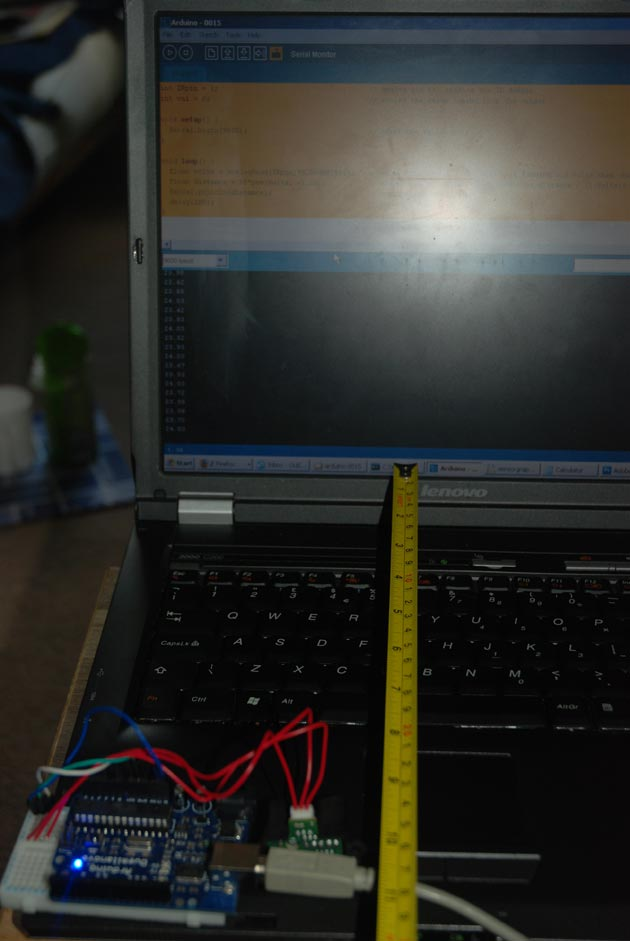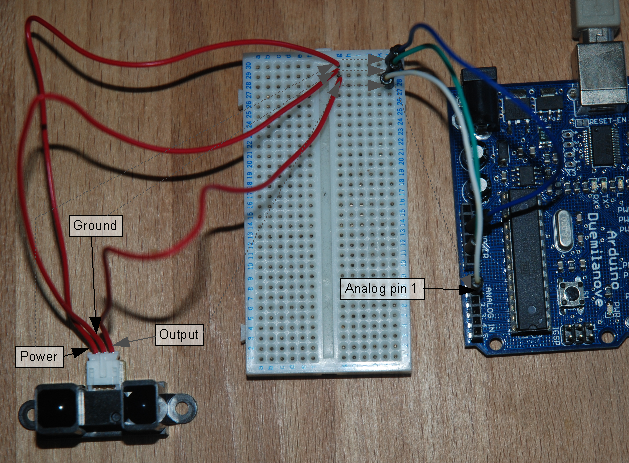# Arduino – Using a Sharp IR Sensor for Distance Calculation

Well, looks like my sonar sensor (SRF05) is a just a tad inaccurate for precise measurement as I found from my radar screen I made (Arduino Radar Sscreen).

So I’ve got hold of a Sharp GP2Y0A02 series infrared distance sensor. It’ll detect and measure anything within a 20-150cm range and it does this by triangulation from where it emits a beam of IR and from when it receives it – this isn’t too important to understand.The hardest bit of this is actually just getting a rough distance value out of it. If we look at the data sheet you’ll see a graph of Volts to Distance and the greater the voltage the shorter the distance. So to measure distance we’ll need to measure the voltage change as the distance changes.

To do this I have to use the analog pins on the Arduino board, now first off because we’re connecting/ reading directly from the sensor we need to convert the digital value that the Arduino’s onboard analog to digital converters will give us. Sounds odd? Well when you use the analog pins it converts what ever arbitary analog value supplied to a byte value which is between 0 and 1023 (1024 variations).

Looking at our graph above from the datasheet, this converted value is of no use to us so we need to convert this value back to the true analog value. How? We take the voltage rating of the power supply and divide by 1024 to give us a value per step. So for instance:

5v/1024 = 0.0048828125

We take this value and multiply by what the sensor sends back to get our voltage reading. The next stage is to work out an equation from the graph on the data sheet to get the theoretical distance from the voltage reading, if we look at the graph between 20 and 150cm you can see that its exponential.

To get our distance on the graph I came up with:

1/Volts * 65

I got 65 by taking the distance on the graph and dividing that by 1/Volts. So now we know what to multiply our voltage results by to get the distance. This is fine if we just had one value to read or if this was a linear graph, in that the change in voltage was always the same amount of distance.

For the exponential change we have to turn our value now into an exponent, a fancy way of saying x to the power of y. So our formula for distance from voltage reading is now something like:

distance = (Volts x)*65.

Since our graph is a decaying exponential the value of x will be a fraction – to write this as a exponent we have to use a minus number e.g. -1. The exponent -1 is the equivalent of the fraction 1/2 which is 0.5 as a decimal.

Now through trial and error I programmed this into my Arduino and changed the exponent until my readings became accurate(ish) I started at -1 and by co-incidence the next value I tried was -1.1 which is 11/10 as a fraction, 0.65 as a decimal and oddly enough 1/100th of my distance ration, 65!

READ  Arduino Prototyping Shield on the cheapAnyway its not spot on but this seems to work for my sensor, for any other Sharp IR sensor, first work out the distance ratio from the graph and then try changing the exponential until you get accurate results. If anyone can show me the equations for doing this that’d be great as I only did this through trial and error.

For more detail: Arduino – Using a Sharp IR Sensor for Distance Calculation

#### This Post / Project can also be found using search terms:

• using two IR sensor to calculate distance arduino protues
• arduino using a sharp ir sensor for distance calculation
• Arduino – Using a Sharp IR
• distance calculating using ir